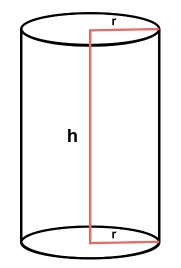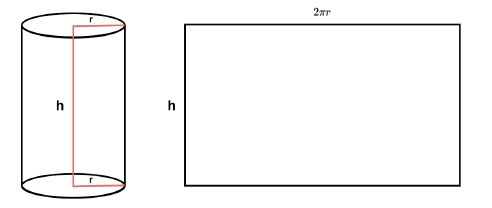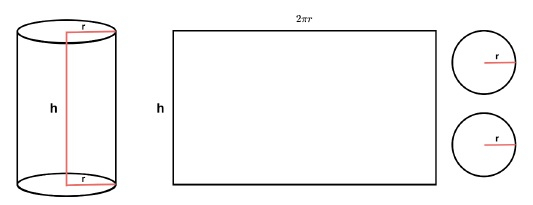# C++ Program to calculate the volume and area of the Cylinder

The cylinder is a tube-like 3D object whose base is a circle and has a certain height. The volume of a cylinder is actually how much space it is taking in the space. In this article, we shall cover a few techniques to calculate the volume and the surface area of a cylinder in C++.

A cylinder has two basic parameters. The height ℎ and the radius of its bases 𝑟. The surface area of a cylinder can have two variations. Only the outer curved surface (for hollow and both side open cylinder) and area with the curved surface with two plane faces (circular shape).

## The volume of a Cylinder

To calculate the volume of a cylinder, we need two inputs, the height ℎ, and the radius 𝑟. The formula for the volume is like below

$$Volume\:=\:\pi\:r^2*h$$Here $\pi\:r^2$ is the area of the base and multiplying this with h will return the entire volume. Now let us see the algorithm and implementation for the same.

### Algorithm

• Surface_area := $\pi\:r^2$
• Volume := Surface_area * h
• Return Volume

### Example

#include <iostream>
using namespace std;

float solve( float h, float r ) {
float volume;
float sa = 3.14159 * r * r;
volume = sa * h;
return volume;
}
int main() {
cout << "Volume of a cylinder with height h = 5 cm, radius r = 2.5 cm, is " << solve( 5, 2.5 ) << " cm^3" << endl;
cout << "Volume of a cylinder with height h = 25 in, radius r = 15 in, is " << solve( 25, 15 ) << " in^3" << endl;
}


### Output

Volume of a cylinder with height h = 5 cm, radius r = 2.5 cm, is 98.1747 cm^3
Volume of a cylinder with height h = 25 in, radius r = 15 in, is 17671.4 in^3


## Curved Surface Area of a Cylinder

The area of only the curved surface can be calculated if we unfold the cylinder like a rectangular sheet where the width is the same as ℎ and the width is the same as 2𝜋𝑟 (perimeter of the circle). In the following diagram, we have shown how the curved surface can be unfolded to represent this as a rectangle.

$$Curved\:Surface\:Area\:=\:2\pi\:r*h$$The following algorithm is to calculate the curved surface area for a given cylinder −

### Algorithm

• perimeter := $2/pi/:r$
• area := perimeter * h
• Return area

### Example

#include <iostream>
using namespace std;
float solve( float h, float r ) {
float area;
float perimeter = 2 * 3.14159 * r;
area = perimeter * h;
return area;
}
int main() {
cout << "Curved Surface Area of a cylinder with height h = 5 cm, radius r = 2.5 cm, is " << solve( 5, 2.5 ) << " cm^3" << endl;
cout << "Curved Surface Area of a cylinder with height h = 25 in, radius r = 15 in, is " << solve( 25, 15 ) << " in^3" << endl;
}


### Output

Curved Surface Area of a cylinder with height h = 5 cm, radius r = 2.5 cm, is 78.5397 cm^3
Curved Surface Area of a cylinder with height h = 25 in, radius r = 15 in, is 2356.19 in^3


## Complete Surface Area of a Cylinder

The complete surface area consists of a curved surface area with two circular sides of the cylinder. So we need to add them with the curved surface area to get the final area.

$$Curved\:Surface\:Area\:=\:2\pi\:r*h$$

$$Circle\:Area\:=\:\pi\:r\:^\:2$$

$$Complete\:Area\:=\:(Curved\:Surface\:Area)\:+\:2(Circle\:Area)$$

Since there are two circular plane surfaces, we have multiplied the Circle Area by 2 before adding. The idea is described in the following figure. Like the previous figure, the rectangular sheet is also there, and two circular faces are added. In the following algorithm, we are calculating the same.The algorithm is shown below −

### Algorithm

• perimeter := $2\pi\:r$
• circle_area :=$\pi\:r^2$
• area := (perimeter * h) + 2 * circle_area
• Return area

### Example

#include <iostream>
using namespace std;

float solve( float h, float r ) {
float area;
float perimeter = 2 * 3.14159 * r;
float circle_area = 3.14159 * r * r;
area = (perimeter * h) + (2 * circle_area);
return area;
}
int main() {
cout << "Complete Area of a cylinder with height h = 5 cm, radius r = 2.5 cm, is " << solve( 5, 2.5 ) << " cm^3" << endl;
cout << "Complete Area of a cylinder with height h = 25 in, radius r = 15 in, is " << solve( 25, 15 ) << " in^3" << endl;
}


### Output

Complete Area of a cylinder with height h = 5 cm, radius r = 2.5 cm, is 117.81 cm^3
Complete Area of a cylinder with height h = 25 in, radius r = 15 in, is 3769.91 in^3


## Conclusion

To calculate the volume and the area of a cylinder, we need two parameters. The base radius and the height of the cylinder. To get the overall volume, at first the base area needs to be calculated, then multiply it with the height to get the actual volume. The area of a cylinder has two forms. One is only the curved surface area, and another one is the complete area where we consider the base plane circular face as well. To calculate this, we can unwrap a cylinder into a rectangular sheet with a height h and length same as the perimeter of the circle, then the area of this rectangle is the area of the curved surface. To get the complete area we need to add two plane surface areas along with the curved surface area. In the above implementations, we have shown the C++ programs to calculate the same with a few example inputs. For each case, only two inputs h and r are needed.